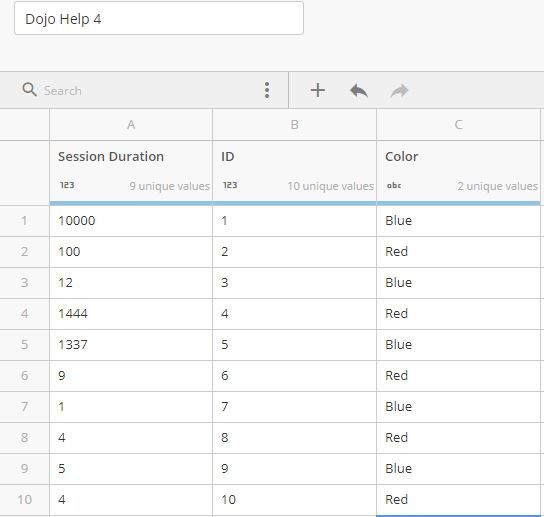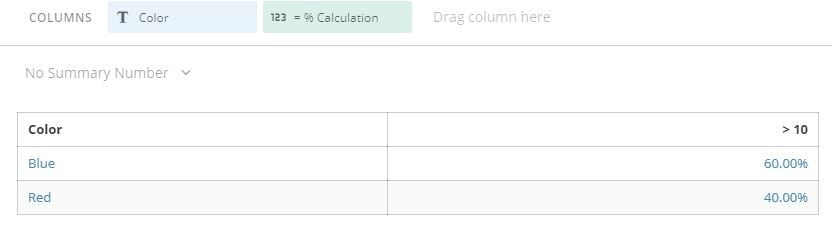# Domo Beast Mode/Data Flow

Hello,

I currently have a column in my data set that is numerical called "session duration" with values ranging from 0 to 45,000 (in seconds). I want to be able to create a new column that does value mapping for values > 10 to be identified as "Greater than 10" and values < 10 to be identified as "Less than 10". Currently, I am able to do this using this formula:

(CASE
WHEN `Session Duration` > 10 THEN 'GTHEN'
ELSE 'LTHEN'
END)

Now I would like to use this calculation to return the  % of total for each the count of category, so a pivot table would show me the % of total of gthen/lthen per month of the year.

• In addition to what @NewsomSolutions said, if doing it in a table or pivote table you might be able to leverage a formula like this.

SUM((CASE
WHEN `Session Duration` > 10 THEN 1
ELSE 0
END))

/

COUNT(`ID`)

I'm not sure exactly what sort of "Categories" you're talking about because you don't really mention it but here's an example where we have durations stats and each row pertains to a color. The calculation shows that when the category is "Blue" the session duration is over 10 60% of the time (3/5), when the category is "Red" the session duration is over 10 40% of the time (2/5).

Test Dataset